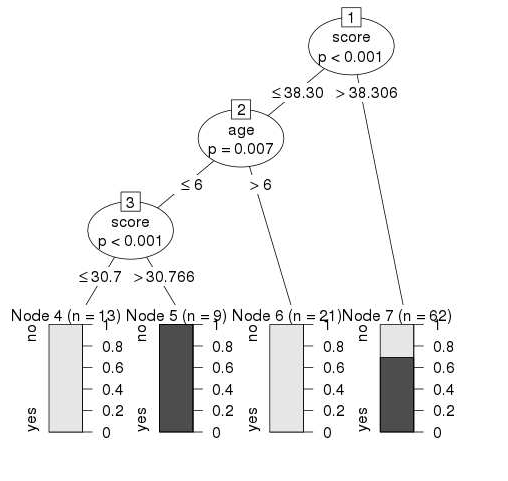# R - Decision Tree

Decision tree is a graph to represent choices and their results in form of a tree. The nodes in the graph represent an event or choice and the edges of the graph represent the decision rules or conditions. It is mostly used in Machine Learning and Data Mining applications using R.

Examples of use of decision tress is − predicting an email as spam or not spam, predicting of a tumor is cancerous or predicting a loan as a good or bad credit risk based on the factors in each of these. Generally, a model is created with observed data also called training data. Then a set of validation data is used to verify and improve the model. R has packages which are used to create and visualize decision trees. For new set of predictor variable, we use this model to arrive at a decision on the category (yes/No, spam/not spam) of the data.

The R package "party" is used to create decision trees.

## Install R Package

Use the below command in R console to install the package. You also have to install the dependent packages if any.

```install.packages("party")
```

The package "party" has the function ctree() which is used to create and analyze decison tree.

### Syntax

The basic syntax for creating a decision tree in R is −

```ctree(formula, data)
```

Following is the description of the parameters used −

• formula is a formula describing the predictor and response variables.

• data is the name of the data set used.

### Input Data

We will use the R in-built data set named readingSkills to create a decision tree. It describes the score of someone's readingSkills if we know the variables "age","shoesize","score" and whether the person is a native speaker or not.

Here is the sample data.

```# Load the party package. It will automatically load other
# dependent packages.
library(party)

# Print some records from data set readingSkills.
```

When we execute the above code, it produces the following result and chart −

```  nativeSpeaker   age   shoeSize      score
1           yes     5   24.83189   32.29385
2           yes     6   25.95238   36.63105
3            no    11   30.42170   49.60593
4           yes     7   28.66450   40.28456
5           yes    11   31.88207   55.46085
6           yes    10   30.07843   52.83124
...............................
...............................
```

### Example

We will use the ctree() function to create the decision tree and see its graph.

```# Load the party package. It will automatically load other
# dependent packages.
library(party)

# Create the input data frame.

# Give the chart file a name.
png(file = "decision_tree.png")

# Create the tree.
output.tree <- ctree(
nativeSpeaker ~ age + shoeSize + score,
data = input.dat)

# Plot the tree.
plot(output.tree)

# Save the file.
dev.off()
```

When we execute the above code, it produces the following result −

```null device
1

Attaching package: ‘zoo’

The following objects are masked from ‘package:base’:

as.Date, as.Date.numeric